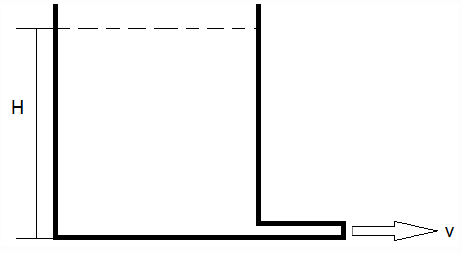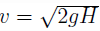Courses

# Test: Types of Fluid Flow

## 10 Questions MCQ Test Fluid Mechanics | Test: Types of Fluid Flow

Description
This mock test of Test: Types of Fluid Flow for Civil Engineering (CE) helps you for every Civil Engineering (CE) entrance exam. This contains 10 Multiple Choice Questions for Civil Engineering (CE) Test: Types of Fluid Flow (mcq) to study with solutions a complete question bank. The solved questions answers in this Test: Types of Fluid Flow quiz give you a good mix of easy questions and tough questions. Civil Engineering (CE) students definitely take this Test: Types of Fluid Flow exercise for a better result in the exam. You can find other Test: Types of Fluid Flow extra questions, long questions & short questions for Civil Engineering (CE) on EduRev as well by searching above.
QUESTION: 1

### Which method is used exclusively in fluid mechanics?

Solution:

Explanation: In Fluid Mechanics, the matter of concern is the general state of motion at various points in the fluid system (as in Eulerian approach) rather than the motion of each particle (as in Lagrangian approach). Hence, the Eulerian method is extensively used in Fluid Mechanics.

QUESTION: 2

### A beaker contains water up to a certain height as shown. If the water is allowed to get discharged through a small pipe (of a uniform diameter), what type of flow will it be in the pipe?Solution:

Explanation: The velocity in which the water is discharged with a velocityWith time as the water gets discharged,v decreases as H decreases. Hence, it will be an unsteady flow.
According to the continuity equation, ρAV =constant, where ρ= density, A= cross-sectional area of flow, V = velocity of flow. Since water is treated as an incompressible liquid (ρ =constant) and the pipe has a uniform diameter (A =constant) at a given instant, V will remain constant throughout the whole cross-section of the pipe. Hence, it will be a uniform flow.

QUESTION: 3

### What type of flow can be taken for granted in a pipe of a uniform cross-section?

Solution:

Explanation: According to the continuity equation, ρAV =constant, where ρ= density, A= cross-sectional area of flow, V = velocity of flow. For a pipe of a uniform cross-section, no matter what the rate of flow is, the velocity of flow inside the pipe will always remain constant. Hence, it’ll always be a uniform flow. It’ll be a steady flow if and only if the water level is maintained at a constant level by supplying water at the same rate as it gets discharged, else the water level will keep decreasing with time leading to an unsteady flow.

QUESTION: 4

Can the flow inside a nozzle be steady and uniform?

Solution:

Explanation: According to the continuity equation, ρAV =constant, where ρ= density, A= cross-sectional area of flow, V = velocity of flow. For a nozzle, the area gradually decreases towards it’s exit. Thus, no matter what the rate of flow is, the velocity of flow at the nozzle exit will always be greater than that at it’s entrance. Hence, it’ll always be an unsteady flow. It can be a steady flow if and only if the water level is maintained at a constant level by supplying water at the same rate as it gets discharged, else the water level will keep decreasing with time leading to an unsteady flow.

QUESTION: 5

Which of the following statements is true regarding one and two-dimensional flows?

Solution:

Explanation: The flow inside a pipe can be described by the cylindrical co-ordinate system (r; θ; z), where r is in the radial direction, θ in the angular direction and z in the axial direction. For a circular cross-sections, the flow can be taken to be independent of θ. Hence, it can be taken aa a two-dimensional flow. Again if aerage flow parameters are considered to account for the variation in the radial direction, the flow can be taken as an one-dimensional flow.

QUESTION: 6

Which of the following is true?

Solution:

Explanation: When a torque is applied to a fluid particle, it undergoes a rotation. Thus, the rotation of a fluid particle will alwayds be associated with shear stress. Shear stress is in turn dependent on the viscosity. Hence, rotational flow occurs where the viscosity effects are predominant. Since, viscosity effects are predominant inside the blundary layer, the flow will be rotational in this region. However, outside the boundary layer, the viscosity effects are negligible. Hence, flow can be treated as irrotational outside the boundary layer.

QUESTION: 7

Which of the following is true?

Solution:

Explanation: Flows can be characterized as laminar or turbulent on the basis of Reynold’s number Re = ρvd / μ, where ρ is the density, d is the pipe diameter and μ is the viscosity. For Re < 2000, the flow will be laminar and Re > 4000, the ow will be turbulent. For laminar flow, the viscosity effects must be high (μ should be high) as inside the boundary layer. Outside the boundary layer, the viscosity effects are negligible. Hence, the flow will be turbulent.

QUESTION: 8

“The velocity of entrance and exit through a nozzle remains the same.” Is this ever possible?

Solution:

Explanation: According to the continuity equation, ρAV =constant, where ρ= density, A= cross sectional area of flow, V = velocity of flow. If v =constant, ρA =constant. Thus a change is A will mean a change in ρ. Hence, the flow is possible only if the fluid is compressible.

QUESTION: 9

Three pipe flows named as 1,2 and 3 are observed. The Reynold’s number for the three are 100, 1000 and 10000. Which of the flows will be laminar?

Solution:

Flows can be characterized as laminar or turbulent on the basis of Reynold’s number Re = ρvd / μ, where ρ is the density, d is the pipe diameter and μ is the viscosity. For Re < 2000, the flow will be laminar and Re > 4000, the flow will be turbulent. Thus, flow 1 and 2 will be laminar.

QUESTION: 10

Three flows named as 1,2 and 3 are observed. The flow velocities are v1 and v2. If all other geometrical factors remain the same along with the fluid considered, flow is more likely to be laminar?

Solution:

Explanation: Flows can be characterized as laminar or turbulent on the basis of Reynold’s number Re = ρvd / μ, where ρ is the density, d is the pipe diameter and μ is the viscosity. If all other geometrical factors remain the same along with the fluid considered, v1 > v2 implies Re1 > Re2. Thus, flow 2 is more likely to be laminar.